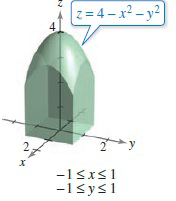Chapter 14, Problem 19RE

Chapter
Section
Textbook Problem

Finding VolumeIn Exercises 17–20, use a double integral to find the volume of the indicated solid.To determine

To calculate: The volume of the solid bounded by z=4x2y2, 1x1 and 1y1 by double integration for the region shown belowExplanation

Given:

The function is z=4x2y2.

The diagram given is:

Formula used:

The volume of a solid when z=f(x,y) is providedby

V=x1x2y1y2f(x,y)dydx

Using the integration formula,

xndx=xn+1n+1

Calculation:

Apply the limits and given function f(x,y)=4x2y2 in the formula of volume:

1111(4x2y2)dydx=11[4yx2yy33]11dx=

Still sussing out bartleby?

Check out a sample textbook solution.

See a sample solution

The Solution to Your Study Problems

Bartleby provides explanations to thousands of textbook problems written by our experts, many with advanced degrees!

Get Started

Find more solutions based on key concepts